# RS Aggarwal Class 7 Solutions Chapter 5 Exponents Ex 5C

In this chapter, we provide RS Aggarwal Solutions for Class 7 Chapter 5 Exponents Ex 5C for English medium students, Which will very helpful for every student in their exams. Students can download the latest RS Aggarwal Solutions for Class 7 Chapter 5 Exponents Ex 5C Maths pdf, free RS Aggarwal Solutions Class 7 Chapter 5 Exponents Ex 5C Maths book pdf download. Now you will get step by step solution to each question.

### RS Aggarwal Solutions for Class 7 Chapter 5 Exponents Ex 5C Download PDF

Question 1.
Solution:
(d)Question 2.
Solution:
(c)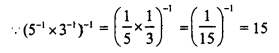Question 3.
Solution:
(c)Question 4.
Solution:
(b)Question 5.
Solution:
(c)Question 6.
Solution:
(b)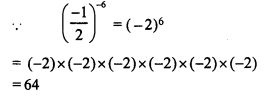Question 7.
Solution:
(b)Question 8.
Solution:
(a)Question 9.
Solution:
(c)Question 10.
Solution:
(b)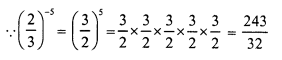Question 11.
Solution:
(b)Question 12.
Solution:
(b)Question 13.
Solution:
(d)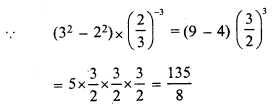Question 14.
Solution:
(a)Question 15.
Solution:
(c)Question 16.
Solution:
(a)Question 17.
Solution:
(c)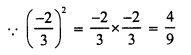Question 18.
Solution:
(b)Question 19.
Solution:
(c)Question 20.
Solution:
(c)
Required number = 10-1 ÷ (-8)-1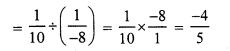Question 21.
Solution:
(c)
The number which is in standard form is 2.156 x 106

All Chapter RS Aggarwal Solutions For Class 7 Maths

—————————————————————————–

All Subject NCERT Exemplar Problems Solutions For Class 7

All Subject NCERT Solutions For Class 7

*************************************************

I think you got complete solutions for this chapter. If You have any queries regarding this chapter, please comment on the below section our subject teacher will answer you. We tried our best to give complete solutions so you got good marks in your exam.

If these solutions have helped you, you can also share rsaggarwalsolutions.in to your friends.